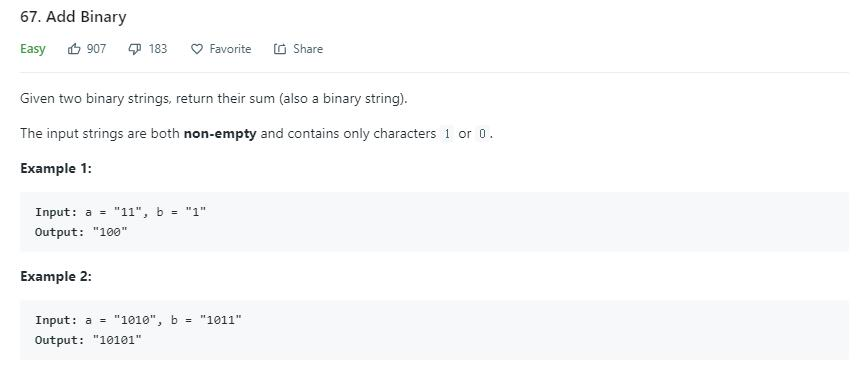# 题目描述（简单难度）# 解法一

public String addBinary(String a, String b) {
StringBuilder ans = new StringBuilder();
int i = a.length() - 1;
int j = b.length() - 1;
int carry = 0;
while (i >= 0 || j >= 0) {
int num1 = i >= 0 ? a.charAt(i) - 48 : 0;
int num2 = j >= 0 ? b.charAt(j) - 48 : 0;
int sum = num1 + num2 + carry;
carry = 0;
if (sum >= 2) {
sum = sum % 2;
carry = 1;
}
ans.insert(0, sum);
i--;
j--;

}
if (carry == 1) {
ans.insert(0, 1);
}
return ans.toString();
}


public String addBinary2(String a, String b) {
char[] charsA = a.toCharArray(), charsB = b.toCharArray();
char[] sum = new char[Math.max(a.length(), b.length()) + 1];
int carry = 0, index = sum.length - 1;
for (int i = charsA.length - 1, j = charsB.length - 1; i >= 0 || j >= 0; i--, j--)         {
int aNum = i < 0 ? 0 : charsA[i] - '0';
int bNum     = j < 0 ? 0 : charsB[j] - '0';

int s = aNum + bNum + carry;
sum[index--] = (char) (s % 2 + '0');
carry = s / 2;
}
sum[index] = (char) ('0' + carry);
return carry == 0 ? new String(sum, 1, sum.length - 1) : new String(sum);
}


public String3 addBinary(String a, String b) {
StringBuilder ans = new StringBuilder();
int i = a.length() - 1;
int j = b.length() - 1;
int carry = 0;
while (i >= 0 || j >= 0) {
int num1 = i >= 0 ? a.charAt(i) - 48 : 0;
int num2 = j >= 0 ? b.charAt(j) - 48 : 0;
int sum = num1 + num2 + carry;
carry = 0;
if (sum >= 2) {
sum = sum % 2;
carry = 1;
}
ans.append(sum);
i--;
j--;

}
if (carry == 1) {
ans.append(1);
}
return ans.reverse().toString();
}